# Algebraic variety, automorphism of an

(diff) ← Older revision | Latest revision (diff) | Newer revision → (diff)
Jump to: navigation, search

An invertible morphism of an algebraic variety (or scheme) into itself. The group of all automorphisms of an algebraic variety, which is usually denoted by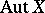, is an important invariant of. Studies of the action of the automorphism group of an algebraic variety on objects functorially connected withsuch as the Picard group; the Chow ring; the-functor, and the cohomology group are a tool used in the study of the varieties themselves. The automorphism group of an algebraic variety is important for the concept of forms (cf. Form) of an algebraic variety. For complete algebraic varieties over the field of complex numbers, the automorphism group is identical with the group of biholomorphic automorphisms.

The structure of the groupis known for a number of simple algebraic varieties. For instance, ifis projective-dimensional space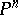over a field, then any one of its automorphisms is a linear projective transformation andbecomes identical with the projective linear group. The automorphism group of an elliptic curve and, in general, of any Abelian variety, is an extension of the groupof automorphisms which preserve the structure of the Abelian variety, by the group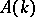of translations in the points of, i.e. the sequence of groups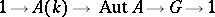is exact. Ifis a smooth, complete algebraic curve of genus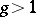, then the groupis finite; an estimate of its order as a function ofis known (cf. Algebraic curve). For automorphisms of surfaces, see Algebraic surface.

In the case of algebraic varieties with an ample canonical or anti-canonical invertible sheaf the automorphism group is an algebraic subgroup of the groupfor some. The automorphism group of a smooth hypersurface of dimensionand degreeis finite .

In the above examples,has the natural structure of an algebraic group, perhaps with an infinite number of connected components; this is also true in the general case .

Families of automorphisms are considered in the modern approach to automorphism groups of algebraic varieties. A family of automorphisms of a varietywith parameter schemeis a set of automorphisms of the product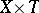that commute with projection onto the second factor; the set of families of automorphisms with parameter schemeis denoted by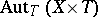. One thus obtains a contravariant functor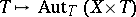. If the varietyis complete, then this functor is locally representable (cf. Representable functor) by an algebraic group scheme with at most a countable number of connected components . A. Grothendieck gave a proof of this fact for projective varieties, and this theorem has been extended to the case of proper flat schemes of morphisms. The scheme representing this functor is not necessarily reduced even ifis a smooth projective surface; however, if the characteristic of the ground field is zero, or ifis a smooth curve or a smooth hypersurface, then the connected component of the unit of this scheme is a variety.

For incomplete varieties the automorphism functor is not always representable in the category of schemes. For an affine variety, the automorphism functor is representable in the category of inductive limits of schemes.

Apart from the simple case of the affine straight line, for the affine spaces only the automorphism group of the affine plane is known. It is a free product of two of its subgroups with as amalgamated subgroup their intersection, viz. the subgroup of linear affine transformations and the subgroup of triangular automorphisms, i.e. transformations of the form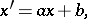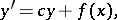where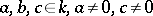, whileis an arbitrary polynomial in, . For the treatment of affine algebraic surfaces transitively acted upon by the automorphism group, see .